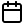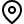## Simultaneously Learning Stochastic and Adversarial Bandits under the Position-Based Model

### Cheng Chen, Canzhe Zhao, Shuai Li

[AAAI-22] Main Track
Abstract: Online learning to rank (OLTR) interactively learns to choose lists of items from a large collection based on certain click models that describe users' click behaviors. Most recent works for this problem focus on the stochastic environment where the item attractiveness is assumed to be invariant during the learning process. In many real-world scenarios, however, the environment could be dynamic or even arbitrarily changing. This work studies the OLTR problem in both stochastic and adversarial environments under the position-based model (PBM). We propose a method based on the follow-the-regularized-leader (FTRL) framework with Tsallis entropy and develop a new self-bounding constraint especially designed for PBM. We prove the proposed algorithm simultaneously achieves $O(\log{T})$ regret in the stochastic environment and $O(m\sqrt{nT})$ regret in the adversarial environment, where $T$ is the number of rounds, $n$ is the number of items and $m$ is the number of positions. We also provide a lower bound of order $\Omega(m\sqrt{nT})$ for adversarial PBM, which matches our upper bound and improves over the state-of-the-art lower bound.

The experiments show that our algorithm could simultaneously learn in both stochastic and adversarial environments and is competitive compared to existing methods that are designed for a single environment.

## Sessions where this paper appears

• #### Poster Session 6Red 2

• #### Poster Session 7Red 2